10.5.1 Right-Looking Deletion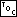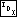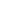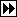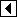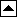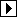```383. <Step 2: Delete RTBST node, right-looking 383> =
if (p->rtbst_rtag == RTBST_THREAD)   {
if (p->rtbst_link != NULL)
{         <Case 1 in right-looking RTBST deletion 384>       }
else       {         <Case 2 in right-looking RTBST deletion 385>       }
} else   {
struct rtbst_node *r = p->rtbst_link;
if (r->rtbst_link == NULL)
{         <Case 3 in right-looking RTBST deletion 386>       }
else       {         <Case 4 in right-looking RTBST deletion 387>       }
}
```

Each of the four cases, presented below, is closely analogous to the same case in TBST deletion.

##### Case 1: p has a right thread and a left child

In this case, node p has a right thread and a left child. As in a TBST, this means that after deleting p we must update the right thread in p's former left subtree to point to p's replacement. The only difference from <Case 1 in TBST deletion 260> is in structure members:

```384. <Case 1 in right-looking RTBST deletion 384> =
struct rtbst_node *t = p->rtbst_link;
while (t->rtbst_rtag == RTBST_CHILD)
```

This code is included in 383.

##### Case 2: p has a right thread and no left child

If node p is a leaf, then there are two subcases, according to whether p is a left child or a right child of its parent q. If dir is 0, then p is a left child and the pointer from its parent must be set to NULL. If dir is 1, then p is a right child and the link from its parent must be changed to a thread to its successor.

In either of these cases we must set q->rtbst_link[dir]: if dir is 0, we set it to NULL, otherwise dir is 1 and we set it to p->rtbst_link. However, we know that p->rtbst_link is NULL, because p is a leaf, so we can instead unconditionally assign p->rtbst_link[dir]. In addition, if dir is 1, then we must tag q's right link as a thread.

If q is the pseudo-root, then dir is 0 and everything works out fine with no need for a special case.

```385. <Case 2 in right-looking RTBST deletion 385> =
if (dir == 1)
```

This code is included in 383.

##### Case 3: p's right child has no left child

Code for this case, where p has a right child r that itself has no left child, is almost identical to <Case 3 in TBST deletion 262>. There is no left tag to copy, but it is still necessary to chase down the right thread in r's new left subtree (the same as p's former left subtree):

```386. <Case 3 in right-looking RTBST deletion 386> =
if (r->rtbst_link != NULL)   {
struct rtbst_node *t = r->rtbst_link;
while (t->rtbst_rtag == RTBST_CHILD)
}
```

This code is included in 383.

##### Case 4: p's right child has a left child

Code for case 4, the most general case, is very similar to <Case 4 in TBST deletion 263>. The only notable difference is in the subcase where s has a right thread: in that case we just set r's left link to NULL instead of having to set it up as a thread.

```387. <Case 4 in right-looking RTBST deletion 387> =
struct rtbst_node *s;

for (;;)   {
if (s->rtbst_link == NULL)
break;

r = s;
}

if (s->rtbst_rtag == RTBST_CHILD)
else   r->rtbst_link = NULL;

if (p->rtbst_link != NULL)   {
struct rtbst_node *t = p->rtbst_link;
while (t->rtbst_rtag == RTBST_CHILD)